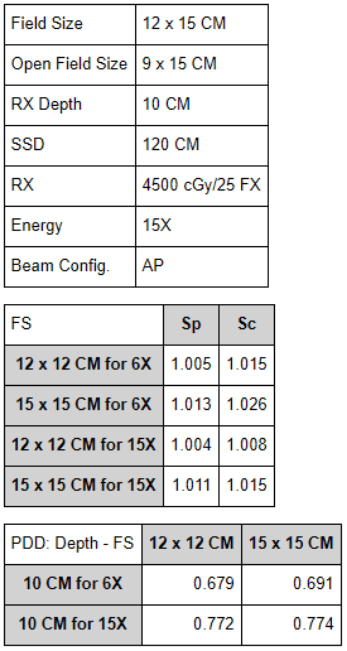The following equations are used for PDD calculations:

1. MU = RX / (k * PDD * SSD factor * OAR * T_f * W_f * Sc * Sp * Maynard’s factor).
• SSD factor: [SCD / (SSD + dmax)] ^2.
• Sc (Collimator Scatter): The scatter from the photons that leave the target source and interact with the collimator to contribute scatter into the center of the field. The field size used to calculate for Sc is the goemetric field size at 100 CM.
• Sp (Phantom Scatter): The scatter that takes place within the patient. The field size used to calculate for Sp is the open field size at the surface of the patient’s skin.
• Maynard’s factor: {[(SSD_2 + dmax) / (SSD_1 + dmax)] ^2} * {[(SSD_1 + depth) / (SSD_2 + depth)] ^2}. A conversion factor used in cases of SSDs greater than 100 CM.
• PDD – percentage depth dose; k – machine calibration factor; OAR – off axis ratio; T_f – tray factor; and W_f – wedge factor. Aside from PDD, the following variables are equal to 1.000 unless otherwise specified.
2. Equivalent Square = 4 * Area (A) / Perimeter (P).The therapist made a mistake and accidentally treated the first 3 fractions using a 6X beam.

• Was this an overdose or an underdose?

15X beams are more penetrative that 6X beams and will require a lesser amount of MUs to deliver the same amount of dose as a 6X beam. Thus, this was an underdose, and the calculations down below will confirm this.

• What was the total dose delivered?

The following are the calculations for the 6X beam:

1. Calculate for SSD factor: {[101.5/(120+1.5)]^2} = 0.698
2. Calulate for Maynard’s factor at SSD of 120 CM for 10 CM depth: {[(120+1.5)/(100+1.5)]^2}*{[(100+10)/(120+10)]^2} = 1.026
3. Calculate for equivalent square field size: 4*(12*15)/(12+12+15+15) = 13.3 x 13.3 CM
4. Interpolate for Sc w/ field size of 13.3 CM: {[(1.026-1.015)/30]*13}+1.015 = 1.020
5. Calculate for open equivalent square field size: 4*(9*15)/(9+9+15+15) = 11.3 x 11.3 CM
6. Calculate for projected open equivalent square field size at SSD of 120 CM: (11.3/100)*120 = 13.6 x 13.6 CM
7. Interpolate for Sp w/ projected open field size of 13.6 CM: {[(1.013-1.005)/30]*16}+1.005 = 1.009
8. Interpolate for PDD at 10 CM depth w/ field size of 13.6 CM: {[(0.691-0.679)/30]*16}+0.679 = 0.685
9. Find MUs delivered by a 6X beam: 180/(0.698*1.026*1.020*1.009*0.685) = 357 MUs

The following are the calculations for the 15X beam:

1. Calculate for SSD factor: {[103/(120+3)]^2} = 0.701
2. Calculate for Maynard’s factor at SSD of 120 CM for 10 CM depth: {[(120+3)/(100+3)]^2}*{[(100+10)/(120+10)]^2} = 1.021
3. Interpolate for Sc w/ field size of 13.3 CM: {[(1.011-1.004)/30]*16}+1.004 = 1.011
4. Interpolate for Sp w/ projected open field size of 13.6 CM: {[(1.013-1.005)/30]*16}+1.005 = 1.008
5. Interpolate for PDD at 10 CM depth w/ field size of 13.6 CM: {[(0.774-0.772)/30]*16}+0.772 = 0.773
6. Find MUs delivered by a 15X beam: 180/(0.701*1.021*1.011*1.008*0.773) = 319 MUs

319 MUs are required to deliver 180 cGy on a 15X beam. Whereas, 357 MUs are required to deliver 180 cGy on a 6X beam. In this problem, 319 MUs intended for a 15X beam were dialed onto a 6X beam for 3 fractions resulting in an underdose.

1. Calculate for dose delivered by 319 MUs on the 6X beam: (180/357)*319 = 160.84 cGy
2. Calculate for dose delivered by the 6X beam: 160.84*3 = 482.52 cGy
3. Calculate for dose delivered by the 15X beam: 180*22 = 3960.00 cGy

Find the total dose delivered: 482.52+3960.00 = 4442.52 cGy